# SSAT Upper Level Math : Coordinate Geometry

## Example Questions

← Previous 1 3 4 5 6 7 8 9 36 37

### Example Question #1 : How To Find The Equation Of A Line

Given the graph of the line below, find the equation of the line.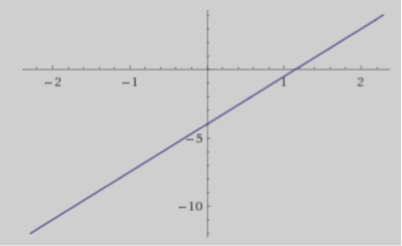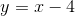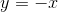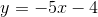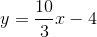Explanation:

To solve this question, you could use two points such as (1.2,0) and (0,-4) to calculate the slope which is 10/3 and then read the y-intercept off the graph, which is -4.

### Example Question #1 : How To Find The Equation Of A Line

What line goes through the points (0, 6) and (4, 0)?

y = 2/3x –6

y = 2/3 + 5

y = 1/5x + 3

y = –3/2 – 3

y = –3/2x + 6

y = –3/2x + 6

Explanation:

P1 (0, 6) and P2 (4, 0)

First, calculate the slope:  m = rise ÷ run = (y2 – y1)/(x– x1), so m = –3/2

Second, plug the slope and one point into the slope-intercept formula:

y = mx + b, so 0 = –3/2(4) + b and b = 6

Thus, y = –3/2x + 6

### Example Question #1 : How To Find The Equation Of A Line

What line goes through the points (1, 3) and (3, 6)?

–2x + 2y = 3

–3x + 2y = 3

2x – 3y = 5

4x – 5y = 4

3x + 5y = 2

–3x + 2y = 3

Explanation:

If P1(1, 3) and P2(3, 6), then calculate the slope by m = rise/run = (y2 – y1)/(x2 – x1) = 3/2

Use the slope and one point to calculate the intercept using y = mx + b

Then convert the slope-intercept form into standard form.

### Example Question #4 : Coordinate Geometry

What is the slope-intercept form of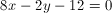?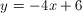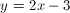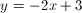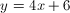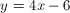Explanation:

The slope intercept form states that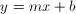. In order to convert the equation to the slope intercept form, isolate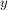on the left side: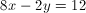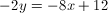### Example Question #5 : Coordinate Geometry

A line is defined by the following equation: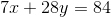What is the slope of that line?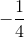Explanation:

The equation of a line is

y=mx + b where m is the slope

Rearrange the equation to match this:

7x + 28y = 84

28y = -7x + 84

y = -(7/28)x + 84/28

y = -(1/4)x + 3

m = -1/4

### Example Question #1 : Other Lines

If the coordinates (3, 14) and (5, 15) are on the same line, what is the equation of the line?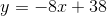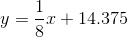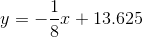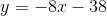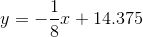Explanation:

First solve for the slope of the line, m using y=mx+b

m = (y2 – y1) / (x2 – x1)

= (15  14) / (5 3)

= (1 )/( 8)

=1/8

y = (1/8)x + b

Now, choose one of the coordinates and solve for b:

14 = (1/8)3 + b

14 = 3/8 + b

b = 14 + (3/8)

b = 14.375

y = (1/8)x + 14.375

### Example Question #2 : Other Lines

What is the equation of a line that passes through coordinates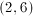and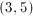?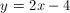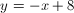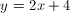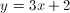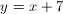Explanation:

Our first step will be to determing the slope of the line that connects the given points.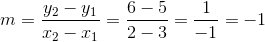Our slope will be. Using slope-intercept form, our equation will be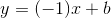. Use one of the give points in this equation to solve for the y-intercept. We will use.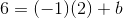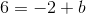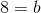Now that we know the y-intercept, we can plug it back into the slope-intercept formula with the slope that we found earlier.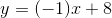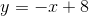### Example Question #1 : Coordinate Geometry

Which of the following equations does NOT represent a line?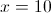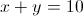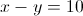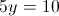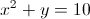Explanation:

The answer is.

A line can only be represented in the form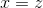or, for appropriate constants,, and. A graph must have an equation that can be put into one of these forms to be a line.represents a parabola, not a line. Lines will never contain anterm.

### Example Question #61 : Coordinate Geometry

Let y = 3x – 6.

At what point does the line above intersect the following: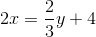(–5,6)

(–3,–3)

(0,–1)

They do not intersect

They intersect at all points

They intersect at all points

Explanation:

If we rearrange the second equation it is the same as the first equation. They are the same line.

### Example Question #1 : Other Lines

A line has a slope ofand passes through the point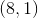. Find the equation of the line.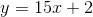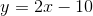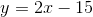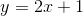Explanation:

In finding the equation of the line given its slope and a point through which it passes, we can use the slope-intercept form of the equation of a line:, whereis the slope of the line andis its-intercept.

Plug the given conditions into the equation to find the-intercept.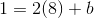Multiply: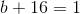Subtractfrom each side of the equation: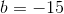Now that you have solved for, you can write out the full equation of the line:← Previous 1 3 4 5 6 7 8 9 36 37

### All SSAT Upper Level Math Resources